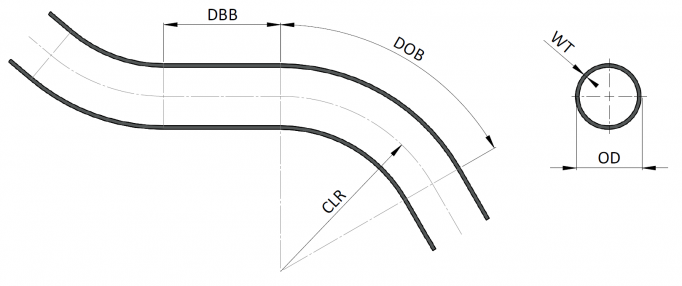Basic Bending Parameters

OD - outer diameter of tube
WT - wall thicknessUsing a variety of parameters, we can judge the difficulty of the bend and suggest the best set of tools for a given application.

Wall Factor (WF) - the relative thickness of the wall. This is the relationship of the outside diameter and the thickness of the walls of the tubes. The resulting value then determines whether it is a thin-walled or a thick-walled tube. Generally we can say that tubes with a relative WT below 10 are considered to be strong walled (thick walled) and conversely with a relative WT higher than 40 are thin walled.

Wall Factor (WF) = the outside diameter OD / thickness of the walls WT.

Note: In some literature on the subject there is the inverted ratio calculation:
Relative wall thickness = wall thickness / external bend radius, however, it is really the same thing reversed.

"D" of bend - or so-called relative bend radius. This parameter determines the character of the bend and whether it is a bend on a large radius or a bend on a small radius.

"D" of bend = Central line radius CLR / Outer diameter of tube OD

Difficulty of bending depends on both parameters at the same time.

There is a practical demonstration of this calculation and their effect on your choice of tool in the Choice of Tools section.

Other parameters that are linked directly with the shape and bend also affect the difficulty bending and the selection of the appropriate tools.

DOB (Degree of bend) - the angle of bend between straight parts of the tube
DBB (distance between bends) - this is a straight distance between bends

For more complex components containing many bends in different planes, we can use the X,Y,Z coordinates of the tubes with the Center Line Radius (CLR) to simply define both the parameters used above, and the DBB for all bends.